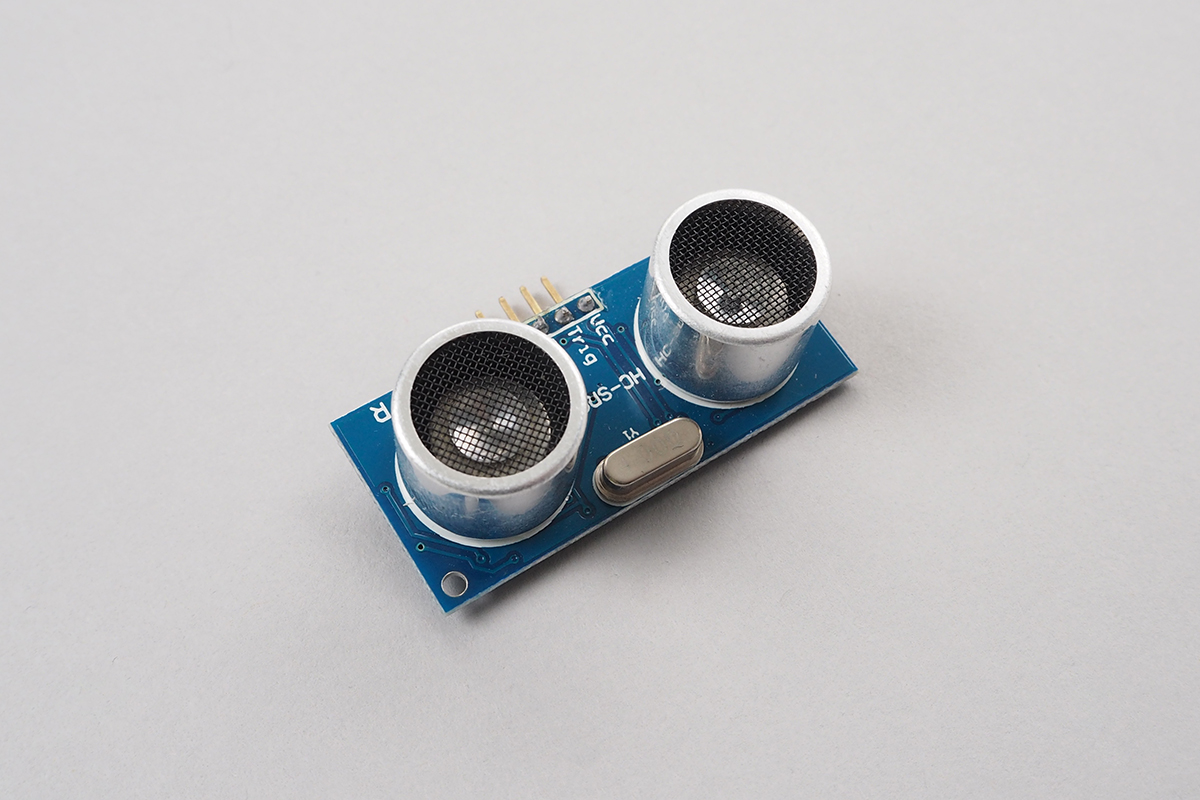# HC-SR04# HC-SR04

Ultrasonic Distance Measurement Unit.## wired(obniz, {vcc, trigger, echo, gnd})``````// Javascript Example
var hcsr04 = obniz.wired("HC-SR04", {gnd:0, echo:1, trigger:2, vcc:3});
hcsr04.measure(function( distance ){
console.log("distance " + distance + " mm")
})
``````

## measure(callback(distance))

The distance is measured and the callback function is called when the measurement is complete.
Default return unit is "mm", which can be changed to inch by calling .unit() function.
If the reflected ultrasonic wave is not received, undefined is returned.

``````// Javascript Example

var hcsr04 = obniz.wired("HC-SR04", {gnd:0, echo:1, trigger:2, vcc:3});
hcsr04.measure(function( distance ){
onsole.log("distance " + distance + " mm")
})
``````

## [await] measureWait()

This is async/await version of measure().

``````// Javascript Example
const hcsr04 = obniz.wired("HC-SR04", {gnd:0, echo:1, trigger:2, vcc:3});
while(true) {
let avg = 0;
let count = 0;
for (let i=0; i<3; i++) { // measure three time. and calculate average
const val = await hcsr04.measureWait();
if (val) {
count++;
avg += val;
}
}
if (count > 1) {
avg /= count;
}
console.log(avg);
await obniz.wait(100);
}
``````

## temp

The speed of ultrasonic depends on temperature.
By default calculation temp is 15 degree(Celsius). Change this to get more accurate result.

``````// Javascript Example
var hcsr04 = obniz.wired("HC-SR04", {gnd:0, echo:1, trigger:2, vcc:3});
hcsr04.temp = 36;
const distance = await hcsr04.measureWait();
console.log("distance " + distance + " mm")
``````

## reset_alltime

Some HC-SR04 needs power reset after measure.
set true to this property to automatic power reset after each measurement.

``````// Javascript Example
var hcsr04 = obniz.wired("HC-SR04", {gnd:0, echo:1, trigger:2, vcc:3});
hcsr04.reset_alltime = true;
const distance = await hcsr04.measureWait();
console.log("distance " + distance + " mm")
``````

## unit(unit)

change unit

1. "mm"(default)
2. "inch"

are available

``````// Javascript Example
var hcsr04 = obniz.wired("HC-SR04", {gnd:0, echo:1, trigger:2, vcc:3});
hcsr04.unit("inch")
hcsr04.measure(function( distance ){
console.log("distance " + distance + " inch")
})
``````

Supported from: obniz.js 3.5.0